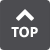##P,Q Parallel Index Cam A conjugate cam used for intermittent feeding applications. The name comes from the parallel configuration of the input and output shafts. Parallel index cams are easy to manufacture compared to roller gear and barrel cams. However, an improper design condition can reduce the load capacity. Pick and Place Unit A unit that picks work up from a certain position, moves to an inserting position and places the work down. Polar Coordinates The coordinates of the position of an object where r represents length and φ represents angle. Polar coordinates are related to the linear Cartesian coordinates as x = rcos (φ) and y = rsin (φ). And Z = Z. Positive Cam A cam that governs both trajectories of the follower. Such cams are the grooved cams and conjugate cams. Pre-load The pressure placed between the cam and follower. The greater the pressure, the better the precision and rigidity; also the more resistance. Pressure Angle The angle that the common normal line of the cam and the follower makes in relation to the moving direction of the follower. Pressure Angle in Dwell Period The pressure angle during a dwell. Necessary when calculating the torque during a dwell. Pressure of Contact Surface The amount of stress that acts upon the curve formed by the contact surfaces of two objects. The pressure of contact surface can be calculated using the Hertz equation. Principle of Virtual Work When a number of forces F1, F2, ... act upon a mass point in a balanced manner, F1 + F2 +... =ΣF = 0. Therefore, the total work δW in respect to any amount of virtual displacement δs is 0. That is δW = ΣF and δs = ΣXδx + ΣYδ + ΣZδz = 0. Virtual work refers to work δW in respect to virtual displacement δs.